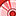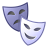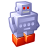# New to Qlik Sense

If you’re new to Qlik Sense, start with this Discussion Board and get up-to-speed quickly.

Announcements
Skip the ticket, Chat with Qlik Support instead for instant assistance.
cancel
Showing results for
Did you mean:Contributor III

## Count if (A value - B Value)>0

I am new for Qlik sense and I get stuck with a count if expression.

I would like to get a distinct count for [PO ID], if "quantity" - "original quantity" <> 0

My expression as below but I am not able to get the result at all.

Count(if(Quantity-[Original Quantity]>0,distinct [PO ID]))

2 Solutions

Accepted SolutionsSpecialist II
Try below expression,

If((sum(Quantity)-sum([Original Quantity]))-=0, Count (Distinct [PO ID]))

Thanks,
Vishal WagholeContributor III
Author

Thanks!

i make some changes on the formula like the following and it works.

if((sum(Quantity))-(sum([Original Quantity]))<0 , Count (DISTINCT [PO ID]))

Thank you!

3 RepliesContributor III

Hi,

You can use the next expression:

if(Quantity-[Original Quantity]>0, Count (DISTINCT [PO ID]))

Regards,Specialist II
Try below expression,

If((sum(Quantity)-sum([Original Quantity]))-=0, Count (Distinct [PO ID]))

Thanks,
Vishal WagholeContributor III
Author

Thanks!

i make some changes on the formula like the following and it works.

if((sum(Quantity))-(sum([Original Quantity]))<0 , Count (DISTINCT [PO ID]))

Thank you!Tags
Community Browser